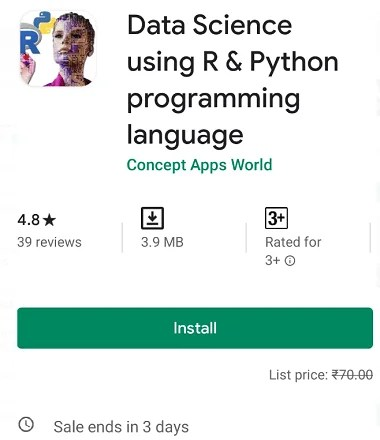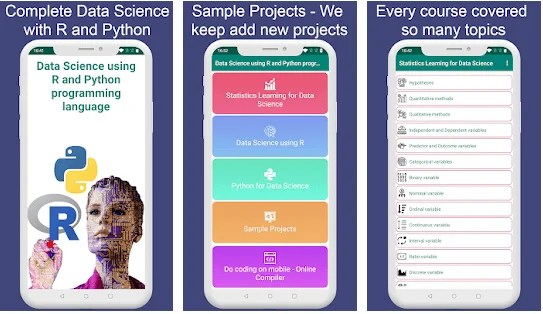# Data Science using R & Python offline tutorial Android App Free

Posted by

Data Science using R & Python offline tutorial is an android learning app available for \$0.99 on Google Playstore. Now the app price reduced to \$0, it’s available free for the next 3 days.

This is a great  Android app for anyone who wants to learn the new R programming language. It’s very useful to learn the basics of data science using Python.

Interested people can reach below link on their Android device and tap the “Install” button.

Data Science, Machine Learning and Artificial intelligence market is on the boom.
Data science is basically converting structured or unstructured data into insight, understanding and knowledge using scientific methods, processes and algorithms.

R and Python are the most common programming languages used in Data Science.

R is a free open-source language used as statistical and visualization software. It can deal with structured (organised) and semi-structured (semi-organised) data.

To learn R for data science, this app covers the following aspects:

•  Introduction
•  Data-Types, variables, and  Operators in R.
•  Conditional, loop and Loop Control Statements
•  R Script & Functions
•  Custom Function and Data Structures
•  Atomic vectors, Matrix, Arrays, Factors, Data Frames and List
•  Import/Export Data – Assign values to the data structure
•  Data Manipulation/Transformation
•  Apply function of Base R
•  dplyr Package

#### For Python, this app covers the following aspects :

• Environment setup and Essentials of Python
•  Introduction and Environment Setup
•  Variable assignment in Python
•  Data Types in Python
•  Data Structure: Tuple, List, Dictionary (Dict) and Set
•  Basic Operator: in, + (plus), * (multiply)
•  Functions
•  Built-in Sequence Function in Python
•  Control Flow Statements: if, elif, else, for Loops and while Loops
•  Exception Handling
• Mathematical Computation with NumPy in Python
•  Types of Arrays
•  Attributes of ndarray
•  Basic Operations
•  Accessing Array Element
•  Copy and Views
•  Universal Functions (ufunc)
•  Shape Manipulation
•  Linear AlgebraStatistics is a crucial part to start learning in in this field.
Terms used in statistics is very strange and hard to understand for beginners, so we tried our best to explain these terms in very easy language for Novice, Intermediate or Advanced level guys in Data Science, Machine Learning, AI field.

The app covers so many terms used in statistics like –

•  Hypotheses
•  Quantitative methods
•  Qualitative methods
•  Independent and Dependent variables
•  Predictor and Outcome variables
•  Categorical variables
•  Binary variable
•  Nominal variable
•  Ordinal variable
•  Continuous variable
•  Interval variable
•  Ratio variable
•  Discrete variable
•  Confounding variables
•  Measurement error
•  Validity and Reliability
•  Two methods of data collection
•  Types of variation
•  Unsystematic variation
•  Systematic variation
•  Frequency distribution
•  Mean, Median and  Mode
•  Dispersion in the distribution of Data
•  Range , Interquartile range and  Quartiles
•  Probability and Standard deviation

A most important advantage of this app that complete material except sample project is available offline, sample project part is online because we keep adding it web-based regular.